Next: 2.6 Grid Refinement Up: 2. Grid Types Previous: 2.4 Demands for the

Subsections

# 2.5 Demands for Finite Elements

Within Finite Elements the coefficients of the matrix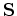shown in (2.8) and (2.9) can be generally expressed as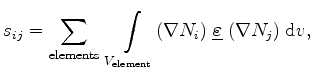(2.19)

respectively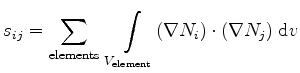(2.20)

for the element volumes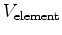. The symbols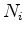and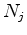denote the Ansatzfunctions of the points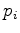and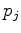. If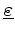is a constant scalar within the tetrahedrons, this in-product has a simple geometrical meaning and the compliance (2.10)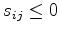(2.21)

leads to an angle criterion for each edge of the mesh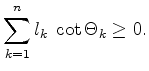(2.22)

 Sum of dihedral angles: Let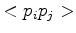be an edge with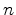adjacent tetrahedra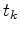. For each tetrahedrontwo planes exist which do not containand which span a dihedral angle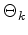. The two planes share an edge with length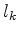. The sum over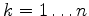of the cotangent ofweighted bymust be greater or equal than zero.

Figure 2.10: Three-dimensional grid criterion for Finite Elements.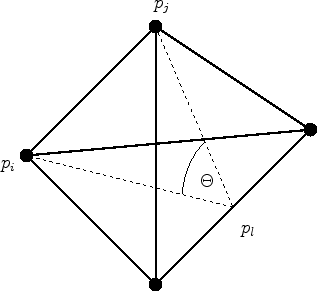Dihedral angle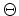of the edgeof a tetrahedron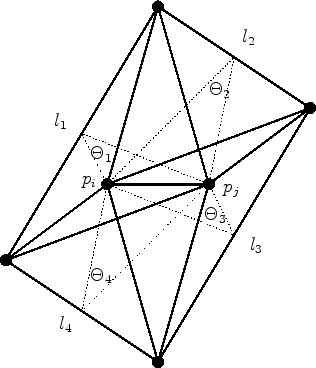Dihedral angles around the edge, arisen from the four tetrahedrons which share the edgeWithin Figure 2.10 the criterion is clarified. In Figure 2.10(a) the dihedral angle of the edgeis shown. It is the angle between the faces which do not contain the edge. Here,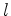is the length of the edge opposing to. In Figure 2.10(b) all the tetrahedrons connected toare shown. Each of the participating tetrahedronsspans its own dihedral angleto.

## 2.5.1 Two-Dimensional Criterion

In two dimensions the criterion (2.22) is also valid. The two triangles connected tocan be considered as tetrahedrons with the same (small) edge, which can be canceled out of the summation, and the dihedral angle of the edgesimplifies to the angle between the two edges of the triangle which do not contain this edge.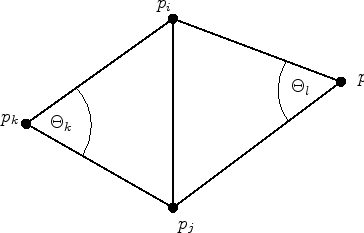The dihedral angle reduces to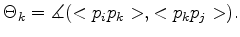(2.23)

As shown in Figure 2.11 in two dimensions exactly two triangles are connected by each edge and (2.22) simplifies to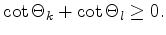(2.24)

With the following transformation and knowing that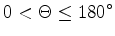or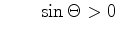(2.25)

we get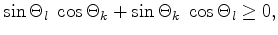(2.26)

which is equivalent to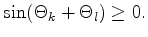(2.27)

Therefore,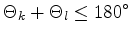(2.28)

is the well-known formulation for two dimensions. This is the same criterion as for Box Integration, given in (2.17). Therefore, the general two-dimensional grid criterion for Finite Boxes and Finite Elements results in the same formulation which is satisfied if the tessellation of the grid points is a Delaunay tessellation. In three dimensions, the criterion for Finite Boxes and Finite Elements differ. Detailed information of the three-dimensional differences can be found in .Next: 2.6 Grid Refinement Up: 2. Grid Types Previous: 2.4 Demands for the

J. Cervenka: Three-Dimensional Mesh Generation for Device and Process Simulation